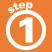Home    |    Teacher    |    Parents    |    Glossary    |    About UsTo divide any number by a fraction:

1. Multiply the number by the reciprocal of the fraction.
2. Simplify the resulting fraction if possible.
3. Check your answer: Multiply the result you got by the divisor and be sure it equals the original dividend.

You can only divide by non-zero fractions.

Click the \$5 bill to see how to divide it by 1/4.

 Homework Help | Pre-Algebra | FractionsEmail this page to a friendSearch·  Definitions·  Reducing fractions·  Adding and subtract-     ing fractions·  Multiplying fractions·  Dividing fractions·  Adding and subtract-     ing mixed numbers·  Multiplying     mixed numbers·  Dividing     mixed numbersFirst Glance In Depth Examples WorkoutDividing fractions